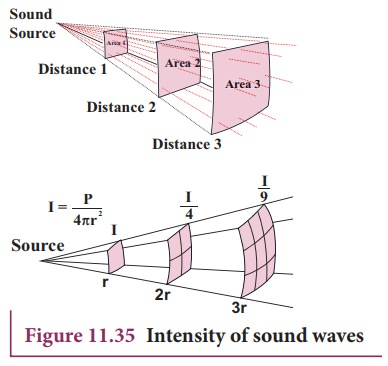Home | | Physics 11th std | Intensity and Loudness of sound

# Intensity and Loudness of sound

Consider a source and two observers (listeners). The source emits sound waves which carry energy.

INTENSITY AND LOUDNESS

Consider a source and two observers (listeners). The source emits sound waves which carry energy. The sound energy emitted by the source is same regardless of whoever measures it, i.e., it is independent of any observer standing in that region. But the sound received by the two observers may be different; this is due to some factors like sensitivity of ears, etc. To quantify such thing, we define two different quantities known as intensity and loudness of sound.

## Intensity of sound

When a sound wave is emitted by a source, the energy is carried to all possible surrounding points. The average sound energy emitted or transmitted per unit time or per second is called sound power. Therefore, the intensity of sound is defined as “the sound power transmitted per unit area taken normal to the propagation of the sound wave ”.For a particular source (fixed source), the sound intensity is inversely proportional to the square of the distance from the source.This is known as inverse square law of sound intensity.

### EXAMPLE 11.23

A baby cries on seeing a dog and the cry is detected at a distance of 3.0 m such that the intensity of sound at this distance is 10-2 W m-2. Calculate the intensity of the baby’s cry at a distance 6.0 m.

### Solution

I1 is the intensity of sound detected at a distance 3.0 m and it is given as 10-2 W m-2. Let I2 be the intensity of sound detected at a distance 6.0 m. Then,

r1 = 3.0 m,

r2 = 6.0 mthe power output does not depend on the observer and depends on the baby. Therefore,I2 = 0.25 × 10-2 W m-2

## Loudness of sound

Two sounds with same intensities need not have the same loudness. For example, the sound heard during the explosion of balloons in a silent closed room is very loud when compared to the same explosion happening in a noisy market. Though the intensity of the sound is the same, the loudness is not. If the intensity of sound is increased then loudness also increases. But additionally, not only does intensity matter, the internal and subjective experience of “how loud a sound is” i.e., the sensitivity of the listener also matters here. This is often called loudness. That is, loudness depends on both intensity of sound wave and sensitivity of the ear (It is purely observer dependent quantity which varies from person to person) whereas the intensity of sound does not depend on the observer. The loudness of sound is defined as “the degree of sensation of sound produced in the ear or the perception of sound by the listener”.

## Intensity and loudness of sound

Our ear can detect the sound with intensity level ranges from 10-2 Wm-2 to 20 W m-2.

According to Weber-Fechner’s law, “loudness (L) is proportional to the logarithm of the actual intensity (I) measured with an accurate non-human instrument”. This means that

L ln I

L = k ln I

where k is a constant, which depends on the unit of measurement. The difference between two loudnesses, L1 and L0 measures the relative loudness between two precisely measured intensities and is called as sound intensity level. Mathematically, sound intensity level isIf k = 1, then sound intensity level is measured in bel, in honour of Alexander Graham Bell. Therefore,However, this is practically a bigger unit, so we use a convenient smaller unit, called decibel. Thus, decibel = 1/10 bel. Therefore, by multiplying and dividing by 10, we getFor practical purposes, we use logarithm to base 10 instead of natural logarithm,### EXAMPLE 11.24

The sound level from a musical instrument playing is 50 dB. If three identical musical instruments are played together then compute the total intensity. The intensity of the sound from each instrument is 10-12 Wm-2

### SolutionI1 = 10-7 Wm-2

Since three musical instruments are played, therefore, Itotal = 3I1 = 3 × 10-7 Wm-2.

Tags : Solved Example Problems | Physics , 11th Physics : UNIT 11 : Waves
Study Material, Lecturing Notes, Assignment, Reference, Wiki description explanation, brief detail
11th Physics : UNIT 11 : Waves : Intensity and Loudness of sound | Solved Example Problems | Physics